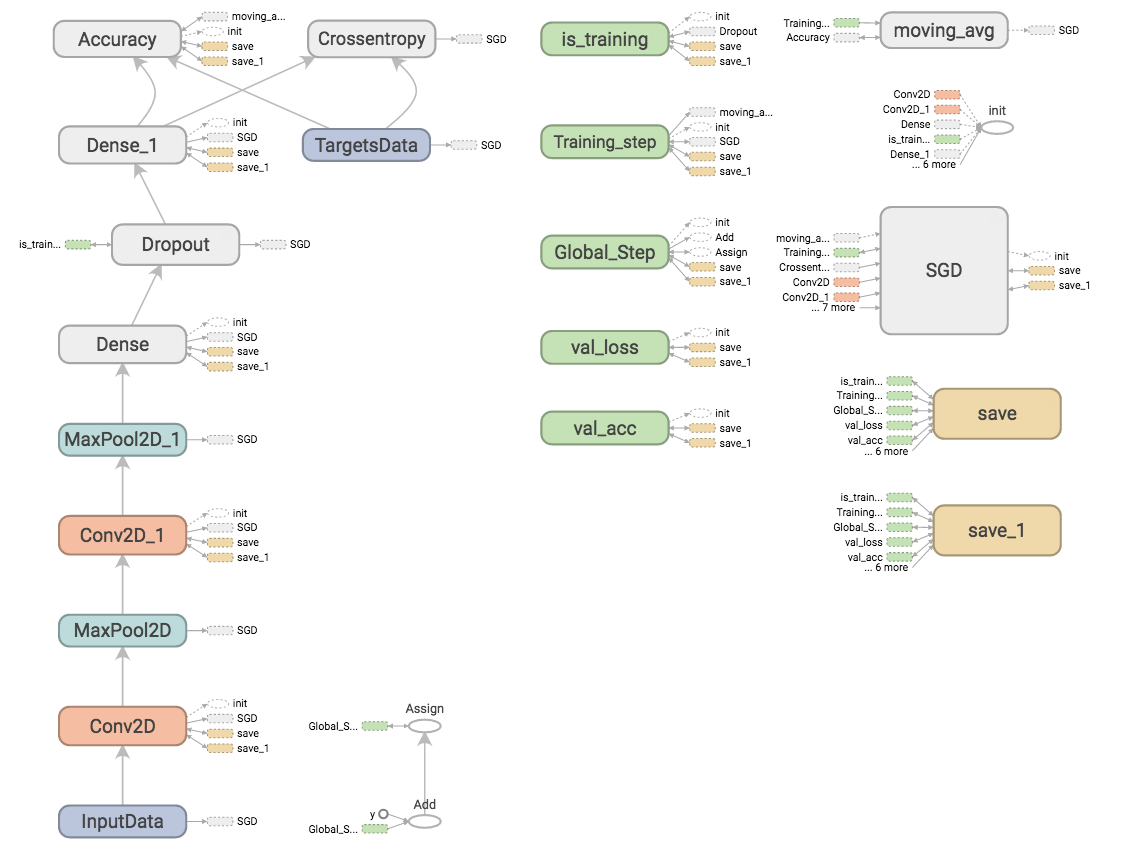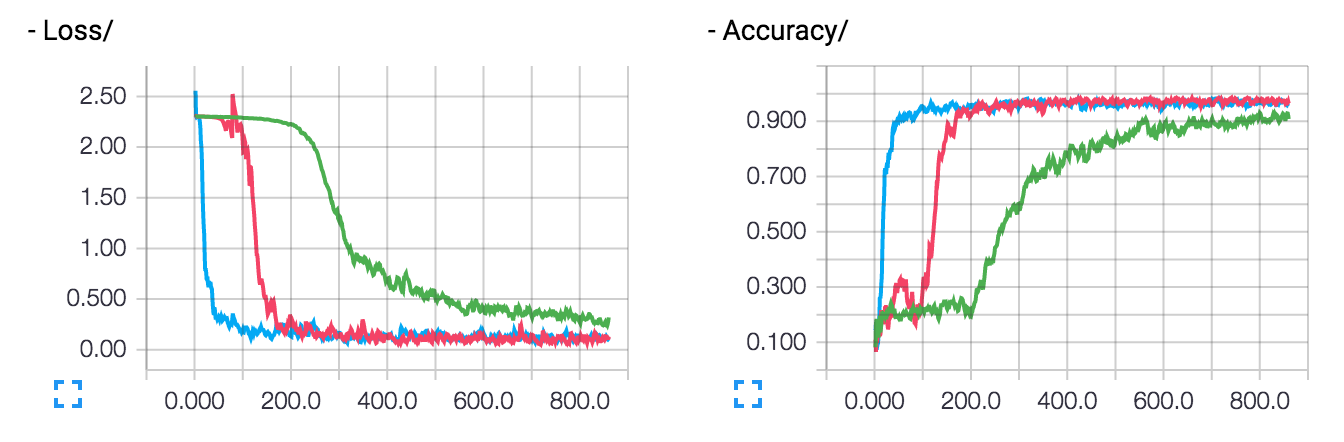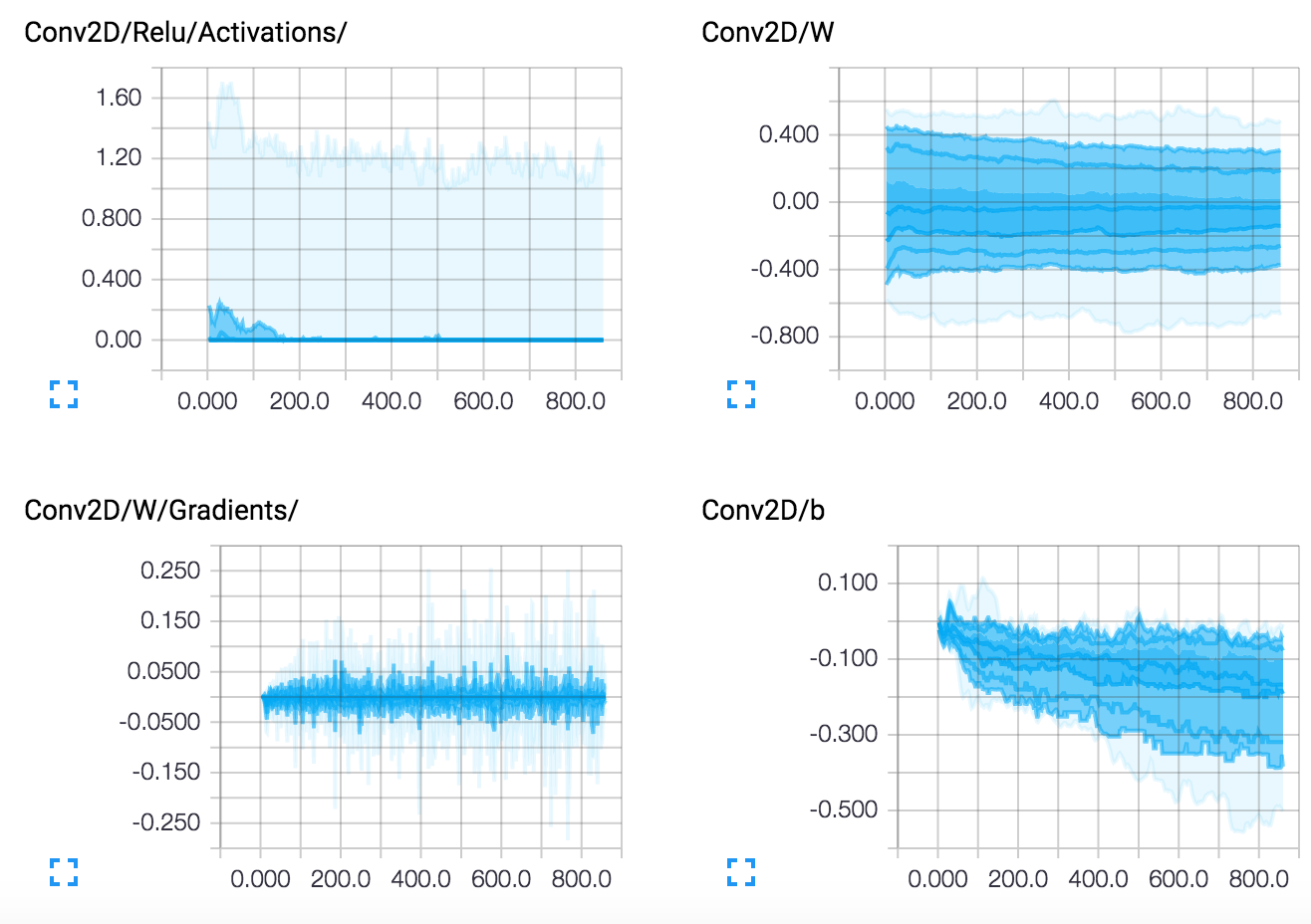# Getting started with TFLearn

Here is a basic guide that introduces TFLearn and its functionalities. First, highlighting TFLearn high-level API for fast neural network building and training, and then showing how TFLearn layers, built-in ops and helpers can directly benefit any model implementation with Tensorflow.

# High-Level API usage

TFLearn introduces a High-Level API that makes neural network building and training fast and easy. This API is intuitive and fully compatible with Tensorflow.

### Layers

Layers are a core feature of TFLearn. While completely defining a model using Tensorflow ops can be time consuming and repetitive, TFLearn brings "layers" that represent an abstract set of operations to make building neural networks more convenient. For example, a convolutional layer will:

• Create and initialize weights and biases variables
• Apply convolution over incoming tensor
• Add an activation function after the convolution
• Etc...

In Tensorflow, writing these kinds of operations can be quite tedious:

``````with tf.name_scope('conv1'):
W = tf.Variable(tf.random_normal([5, 5, 1, 32]), dtype=tf.float32, name='Weights')
b = tf.Variable(tf.random_normal(), dtype=tf.float32, name='biases')
x = tf.nn.conv2d(x, W, strides=[1, 1, 1, 1], padding='SAME')
x = tf.nn.relu(x)
``````

While in TFLearn, it only takes a line:

``````tflearn.conv_2d(x, 32, 5, activation='relu', name='conv1')
``````

Here is a list of all currently available layers:

File Layers
core input_data, fully_connected, dropout, custom_layer, reshape, flatten, activation, single_unit, highway, one_hot_encoding, time_distributed
conv conv_2d, conv_2d_transpose, max_pool_2d, avg_pool_2d, upsample_2d, conv_1d, max_pool_1d, avg_pool_1d, residual_block, residual_bottleneck, conv_3d, max_pool_3d, avg_pool_3d, highway_conv_1d, highway_conv_2d, global_avg_pool, global_max_pool
recurrent simple_rnn, lstm, gru, bidirectionnal_rnn, dynamic_rnn
embedding embedding
normalization batch_normalization, local_response_normalization, l2_normalize
merge merge, merge_outputs
estimator regression

### Built-in Operations

Besides layers concept, TFLearn also provides many different ops to be used when building a neural network. These ops are firstly mean to be part of the above 'layers' arguments, but they can also be used independently in any other Tensorflow graph for convenience. In practice, just providing the op name as argument is enough (such as activation='relu' or regularizer='L2' for conv_2d), but a function can also be provided for further customization.

File Ops
activations linear, tanh, sigmoid, softmax, softplus, softsign, relu, relu6, leaky_relu, prelu, elu
objectives softmax_categorical_crossentropy, categorical_crossentropy, binary_crossentropy, mean_square, hinge_loss, roc_auc_score, weak_cross_entropy_2d
metrics Accuracy, Top_k, R2
initializations zeros, uniform, uniform_scaling, normal, truncated_normal, xavier, variance_scaling
losses l1, l2

Below are some quick examples:

``````# Activation and Regularization inside a layer:
fc2 = tflearn.fully_connected(fc1, 32, activation='tanh', regularizer='L2')
# Equivalent to:
fc2 = tflearn.fully_connected(fc1, 32)
fc2 = tflearn.tanh(fc2)

# Optimizer, Objective and Metric:
reg = tflearn.regression(fc4, optimizer='rmsprop', metric='accuracy', loss='categorical_crossentropy')
# Ops can also be defined outside, for deeper customization:
momentum = tflearn.optimizers.Momentum(learning_rate=0.1, weight_decay=0.96, decay_step=200)
top5 = tflearn.metrics.Top_k(k=5)
reg = tflearn.regression(fc4, optimizer=momentum, metric=top5, loss='categorical_crossentropy')
``````

### Training, Evaluating & Predicting

Training functions are another core feature of TFLearn. In Tensorflow, there are no pre-built API to train a network, so TFLearn integrates a set of functions that can easily handle any neural network training, whatever the number of inputs, outputs and optimizers.

While using TFlearn layers, many parameters are already self managed, so it is very easy to train a model, using `DNN` model class:

``````network = ... (some layers) ...
network = regression(network, optimizer='sgd', loss='categorical_crossentropy')

model = DNN(network)
model.fit(X, Y)
``````

It can also directly be called for prediction, or evaluation:

``````network = ...

model = DNN(network)
model.predict(X)
``````

### Visualization

While writing a Tensorflow model and adding tensorboard summaries isn't very practical, TFLearn has the ability to self managed a lot of useful logs. Currently, TFLearn supports a verbose level to automatically manage summaries:

• 0: Loss & Metric (Best speed).
• 1: Loss, Metric & Gradients.
• 2: Loss, Metric, Gradients & Weights.
• 3: Loss, Metric, Gradients, Weights, Activations & Sparsity (Best Visualization).

Using `DNN` model class, it just requires to specify the verbose argument:

``````model = DNN(network, tensorboard_verbose=3)
``````

Then, Tensorboard can be run to visualize network and performance:

``````\$ tensorboard --logdir='/tmp/tflearn_logs'
``````

GraphLoss & Accuracy (multiple runs)Layers### Weights persistence

To save or restore a model, simply invoke 'save' or 'load' method of `DNN` model class.

``````# Save a model
model.save('my_model.tflearn')
``````

Retrieving a layer variables can either be done using the layer name, or directly by using 'W' or 'b' attributes that are supercharged to the layer's returned Tensor.

``````# Let's create a layer
fc1 = fully_connected(input_layer, 64, name="fc_layer_1")
# Using Tensor attributes (Layer will supercharge the returned Tensor with weights attributes)
fc1_weights_var = fc1.W
fc1_biases_var = fc1.b
# Using Tensor name
fc1_vars = tflearn.get_layer_variables_by_name("fc_layer_1")
fc1_weights_var = fc1_vars
fc1_biases_var = fc1_vars
``````

To get or set the value of these variables, TFLearn models class implement `get_weights` and `set_weights` methods:

``````input_data = tflearn.input_data(shape=[None, 784])
fc1 = tflearn.fully_connected(input_data, 64)
fc2 = tflearn.fully_connected(fc1, 10, activation='softmax')
net = tflearn.regression(fc2)
model = DNN(net)
# Get weights values of fc2
model.get_weights(fc2.W)
# Assign new random weights to fc2
model.set_weights(fc2.W, numpy.random.rand(64, 10))
``````

Note that you can also directly use TensorFlow `eval` or `assign` ops to get or set the value of these variables.

### Fine-tuning

Fine-tune a pre-trained model on a new task might be useful in many cases. So, when defining a model in TFLearn, you can specify which layer's weights you want to be restored or not (when loading pre-trained model). This can be handle with the 'restore' argument of layer functions (only available for layers with weights).

``````# Weights will be restored by default.
fc_layer = tflearn.fully_connected(input_layer, 32)
# Weights will not be restored, if specified so.
fc_layer = tflearn.fully_connected(input_layer, 32, restore='False')
``````

All weights that doesn't need to be restored will be added to tf.GraphKeys.EXCL_RESTORE_VARS collection, and when loading a pre-trained model, these variables restoration will simply be ignored. The following example shows how to fine-tune a network on a new task by restoring all weights except the last fully connected layer, and then train the new model on a new dataset:

### Data management

TFLearn supports numpy array data. Additionally, it also supports HDF5 for handling large datasets. HDF5 is a data model, library, and file format for storing and managing data. It supports an unlimited variety of datatypes, and is designed for flexible and efficient I/O and for high volume and complex data (more info). TFLearn can directly use HDF5 formatted data:

``````# Load hdf5 dataset
h5f = h5py.File('data.h5', 'r')
X, Y = h5f['MyLargeData']

... define network ...

# Use HDF5 data model to train model
model = DNN(network)
model.fit(X, Y)
``````

For an example, see: hdf5.py.

### Data Preprocessing and Data Augmentation

It is common to perform data pre-processing and data augmentation while training a model, so TFLearn provides wrappers to easily handle it. Note also that TFLearn data stream is designed with computing pipelines in order to speed-up training (by pre-processing data on CPU while GPU is performing model training).

``````# Real-time image preprocessing
img_prep = tflearn.ImagePreprocessing()
# Zero Center (With mean computed over the whole dataset)
# STD Normalization (With std computed over the whole dataset)

# Real-time data augmentation
img_aug = tflearn.ImageAugmentation()
# Random flip an image

# Add these methods into an 'input_data' layer
network = input_data(shape=[None, 32, 32, 3],
data_preprocessing=img_prep,
data_augmentation=img_aug)
``````

For more details, see Data Preprocessing and Data Augmentation.

### Scopes & Weights sharing

All layers are built over 'variable_op_scope', that makes it easy to share variables among multiple layers and make TFLearn suitable for distributed training. All layers with inner variables support a 'scope' argument to place variables under; layers with same scope name will then share the same weights.

``````# Define a model builder
def my_model(x):
x = tflearn.fully_connected(x, 32, scope='fc1')
x = tflearn.fully_connected(x, 32, scope='fc2')
x = tflearn.fully_connected(x, 2, scope='out')

# 2 different computation graphs but sharing the same weights
with tf.device('/gpu:0'):
# Force all Variables to reside on the CPU.
with tf.arg_scope([tflearn.variables.variable], device='/cpu:0'):
model1 = my_model(placeholder_X)
# Reuse Variables for the next model
tf.get_variable_scope().reuse_variables()
with tf.device('/gpu:1'):
with tf.arg_scope([tflearn.variables.variable], device='/cpu:0'):
model2 = my_model(placeholder_X)

# Model can now be trained by multiple GPUs (see gradient averaging)
...
``````

### Graph Initialization

It might be useful to limit resources, or assign more or less GPU RAM memory while training. To do so, a graph initializer can be used to configure a graph before run:

``````tflearn.init_graph(set_seed=8888, num_cores=16, gpu_memory_fraction=0.5)
``````

# Extending Tensorflow

TFLearn is a very flexible library designed to let you use any of its component independently. A model can be succinctly built using any combination of Tensorflow operations and TFLearn built-in layers and operations. The following instructions will show you the basics for extending Tensorflow with TFLearn.

### Layers

Any layer can be used with any other Tensor from Tensorflow, this means that you can directly use TFLearn wrappers into your own Tensorflow graph.

``````# Some operations using Tensorflow.
X = tf.placeholder(shape=(None, 784), dtype=tf.float32)
net = tf.reshape(X, [-1, 28, 28, 1])

# Using TFLearn convolution layer.
net = tflearn.conv_2d(net, 32, 3, activation='relu')

# Using Tensorflow's max pooling op.
net = tf.nn.max_pool(net, ksize=[1, 2, 2, 1], strides=[1, 2, 2, 1], padding='SAME')

...
``````

### Built-in Operations

TFLearn built-in ops make Tensorflow graphs writing faster and more readable. So, similar to layers, built-in ops are fully compatible with any TensorFlow expression. The following code example shows how to use them along with pure Tensorflow API.

Here is a list of available ops, click on the file for more details:

File Ops
activations linear, tanh, sigmoid, softmax, softplus, softsign, relu, relu6, leaky_relu, prelu, elu
objectives softmax_categorical_crossentropy, categorical_crossentropy, binary_crossentropy, mean_square, hinge_loss, roc_auc_score, weak_cross_entropy_2d
metrics Accuracy, Top_k, R2
initializations zeros, uniform, uniform_scaling, normal, truncated_normal, xavier, variance_scaling
losses l1, l2

Note: - Optimizers are designed as class and not function, for usage outside of TFlearn models, check: optimizers.

### Trainer / Evaluator / Predictor

If you are using you own Tensorflow model, TFLearn also provides some 'helpers' functions that can train any Tensorflow graph. It is suitable to make training more convenient, by introducing realtime monitoring, batch sampling, moving averages, tensorboard logs, data feeding, etc... It supports any number of inputs, outputs and optimization ops.

TFLearn implements a `TrainOp` class to represent an optimization process (i.e. backprop). It is defined as follow:

``````trainop = TrainOp(net=my_network, loss=loss, metric=accuracy)
``````

Then, all TrainOp can be fed into a `Trainer` class, that will handle the whole training process, considering all TrainOp together as a whole model.

``````model = Trainer(trainops=trainop, tensorboard_dir='/tmp/tflearn')
model.fit(feed_dict={input_placeholder: X, target_placeholder: Y})
``````

While most models will only have a single optimization process, it can be useful for more complex models to handle multiple ones.

``````model = Trainer(trainops=[trainop1, trainop2])
model.fit(feed_dict=[{in1: X1, label1: Y1}, {in2: X2, in3: X3, label2: Y2}])
``````

• For an example, see: trainer.py.

For prediction, TFLearn implements a `Evaluator` class that is working in a similar way as `Trainer`. It takes any network as parameter and return the predicted value.

``````model = Evaluator(network)
model.predict(feed_dict={input_placeholder: X})
``````

To handle networks that have layer with different behavior at training and testing time (such as dropout and batch normalization), `Trainer` class uses a boolean variable ('is_training'), that specifies if the network is used for training or testing/predicting. This variable is stored under tf.GraphKeys.IS_TRAINING collection, as its first (and only) element. So, when defining such layers, this variable should be used as the op condition:

``````# Example for Dropout:
x = ...

def apply_dropout(): # Function to apply when training mode ON.
return tf.nn.dropout(x, keep_prob)

is_training = tflearn.get_training_mode() # Retrieve is_training variable.
tf.cond(is_training, apply_dropout, lambda: x) # Only apply dropout at training time.
``````

To make it easy, TFLearn implements functions to retrieve that variable or change its value:

``````# Set training mode ON (set is_training var to True)
tflearn.is_training(True)
# Set training mode OFF (set is_training var to False)
tflearn.is_training(False)
``````

### Training Callbacks

During the training cycle, TFLearn gives you the possibility to track and interact with the metrics of the training throughout a set of functions given by the Callback interface. To simplify the metrics retrieval, each callback method received a TrainingState which track the state (e.g. : current epoch, step, batch iteration) and metrics (e.g. : current validation accuracy, global accuracy etc..)

Callback methods which relate to the training cycle : - `on_train_begin(training_state)` - `on_epoch_begin(training_state)` - `on_batch_begin(training_state)` - `on_sub_batch_begin(training_state)` - `on_sub_batch_end(training_state, train_index)` - `on_batch_end(training_state, snapshot)` - `on_epoch_end(training_state)` - `on_train_end(training_state)`

#### How to use it:

Imagine you have your own monitor which track all your training jobs and you need to send metrics to it. You can easily do this by creating a custom callback which will get data and send it to the distant monitor. We need to create a CustomCallback and add your logic in the `on_epoch_end` which is called at the end of an epoch.

This will give you something like that:

``````class MonitorCallback(tflearn.callbacks.Callback):
def __init__(self, api):
self.my_monitor_api = api

def on_epoch_end(self, training_state):
self.my_monitor_api.send({
accuracy: training_state.global_acc,
loss: training_state.global_loss,
})

``````

Then you just need to add it on the `model.fit` call

``````

monitorCallback = MonitorCallback(api) # "api" is your API class
model = ...

model.fit(..., callbacks=monitorCallback)

``````

The `callbacks` argument can take a `Callback` or a `list` of callbacks. That's it, your custom callback will be automatically called at each epoch end.

### Variables

TFLearn defines a set of functions for users to quickly define variables.

While in Tensorflow, variable creation requires predefined value or initializer, as well as an explicit device placement, TFLearn simplifies variable definition:

``````import tflearn.variables as vs
my_var = vs.variable('W',
shape=[784, 128],
initializer='truncated_normal',
regularizer='L2',
device='/gpu:0')
``````

### Summaries

When using `Trainer` class, it is also very easy to manage summaries. It just additionally required that the activations to monitor are stored into `tf.GraphKeys.ACTIVATIONS` collection.

Then, simply specify a verbose level to control visualization depth:

``````model = Trainer(network, loss=loss, metric=acc, tensorboard_verbose=3)
``````

Beside `Trainer` self-managed summaries option, you can also directly use TFLearn ops to quickly add summaries to your current Tensorflow graph.

``````import tflearn.helpers.summarizer as s
s.summarize_variables(train_vars=[...]) # Summarize all given variables' weights (All trainable variables if None).
s.summarize_activations(activations=[...]) # Summarize all given activations
s.summarize(value, type) # Summarize anything.
``````

Every function above accepts a collection as parameter, and will return a merged summary over that collection (Default name: 'tflearn_summ'). So you just need to run the last summarizer to get the whole summary ops collection, already merged.

``````s.summarize_variables(collection='my_summaries')
summary_op = s.summarize_activations(collection='my_summaries')
# summary_op is a the merged op of previously define weights, gradients and activations summary ops.
``````

### Regularizers

Add regularization to a model can be completed using TFLearn regularizer. It currently supports weights and activation regularization. Available regularization losses can be found in here. All regularization losses are stored into tf.GraphKeys.REGULARIZATION_LOSSES collection.

``````# Add L2 regularization to a variable
W = tf.Variable(tf.random_normal([784, 256]), name="W")